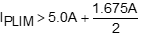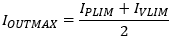# How Overcurrent Protection Works in the MAX77812

### Abstract

The MAX77812 is a quad-phase, high-current, step-down (buck) converter for high-end gaming consoles, VR/AR headsets, DSLR cameras, drones, network switches and routers, and FPGA systems that use multi-core processors. This application note explains the MAX77812 overcurrent protection scheme and provides IPLIM/IVLIM selection guidelines for a given maximum load current.

### Introduction

The MAX77812 provides cycle-by-cycle peak and valley current limit protection by monitoring the current through high- and low-side MOSFETs. The programmable peak current limit (IPLIM) and valley current limit (IVLIM) allows the customer to set the inductor current limit based on the application.

### Overcurrent Protection Scheme

When the output is a short to ground, the output voltage collapses and the average inductor current increases rapidly, which results in hitting the IPLIM threshold. When the fault condition persists, the inductor current can increase the staircase beyond the IPLIM threshold due to the minimum on-time requirement.

In order to address this issue, the MAX77812 introduces the IVLIM threshold. When the inductor current reaches the IPLIM threshold, the high-side MOSFET turns off immediately, allowing the inductor current to discharge its energy through the low-side MOSFET. Until the inductor current falls down to the IVLIM threshold, the high-side MOSFET is not allowed to turn on. Thus, the short circuit current is limited way below the IPLIM threshold.

In the event of a short circuit, the MAX77812 does not terminate its operation. When the output is a short to ground, the output voltage collapses, and a POK interrupt is generated by the MAX77812. Then, an application processor or a microcontroller unit can handle this interrupt signal to recover the system.

Figure 1 shows the overcurrent protection scheme.### IPLIM and IVLIM Selection

The MAX77812 supports the programmable IPLIM and IVLIM thresholds. Each master has its own configuration registers, and there are eight options of IPLIM/IVLIM pairs, as shown in Table 1.

 Mx_ILIM[2:0] in Mx_CFG Registers IPLIM IVLIM ILOADMAX 000b 3.0A 2.0A 2.5A 001b 3.6A 2.4A 3.0A 010b 4.2A 2.8A 3.5A 011b 4.8A 3.2A 4.0A 100b 5.4A 3.6A 4.5A 101b* 6.0A 4.0A 5.0A 110b 6.6A 4.4A 5.5A 111b 7.2A 4.8A 6.0A *POR Default

The following equations and examples are guides to choosing the optimal IPLIM/IVLIM setting. Consider the maximum required load current to determine the IPLIM thresholds. The following equation shows how to decide a proper IPLIM:where ILOADMAX is the maximum load current and ?IL is the inductor current ripple.

Derive the inductor current ripple by using the following equation:For example, a device has the following operating conditions:The calculated inductor current ripple is 1.675A. If the maximum required load current is 5.0A, IPLIM should be higher than 5.84A, as shown by the following calculation:Then, the IPLIM/IVLIM pair can be set to 6.6A/4.4A with some margin.

When the output short occurs, the maximum output current is limited by the IPLIM/IVLIM thresholds, as expressed by the following equation:For example, when the IPLIM/IVLIM thresholds are set to 6.6A/4.4A, the maximum output current is limited to 5.5A.